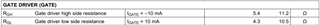If you have a related question, please click the "Ask a related question" button in the top right corner. The newly created question will be automatically linked to this question.

# TPS92691-Q1: The TPS926921 Gate Drive Capability Question

Part Number: TPS92691-Q1

Dear TIer,

May I know the source / sink current of TPS92691-Q1?  How can I calculate the source / sink current through the data sheet parameters?• Hello,

The pull up resistance, to VCC and the pull down resistance to ground is used to calculate the gate drive current at the voltage plateau of the MOSFET used at the current used.

As an example if the MOSFET gate plateau voltage is 4V using the typical VCC value of 7.5V (or worst case of 7.0V) you have 7.5V - 4.0V = 3.5V of drive with 5.4 ohms typical pull up.  This equals 648 mA gate drive at the MOSFET plateau voltage.  If the plateau is 10 nC of energy to 'turn on' the turn on time is approximately 15 nsec (10 nC/648 mA).  Note that the plateau length will depend on VDS voltage.  Turn off will use 4V and 4.3 ohms which will be 930 mA of pull down current for 11 nsec turn-off time.

For loss calculations worst case should be used, VCC = 7.0V and the maximum pull up and pull down resistance.

Best Regards,